Скачать презентацию Chapter 16 Equity Evaluation Models 2015 Mc

0d51e7a8b4c773f35633df17818246ff.ppt

• Количество слайдов: 56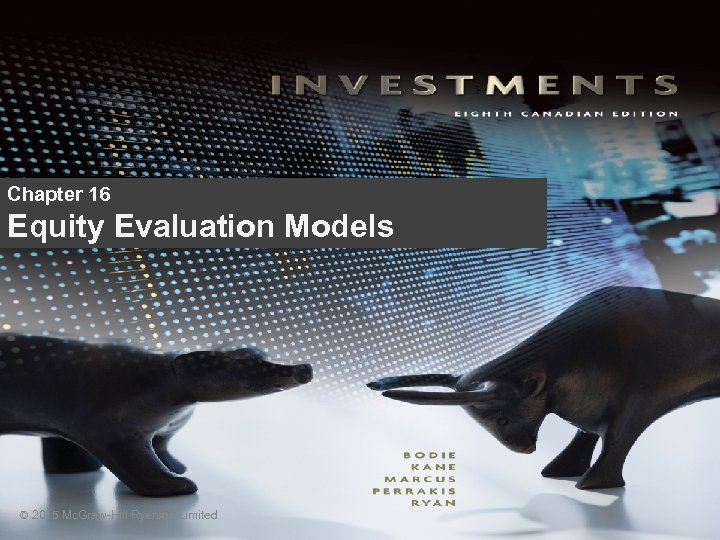Chapter 16 Equity Evaluation Models © 2015 Mc. Graw-Hill Ryerson Limited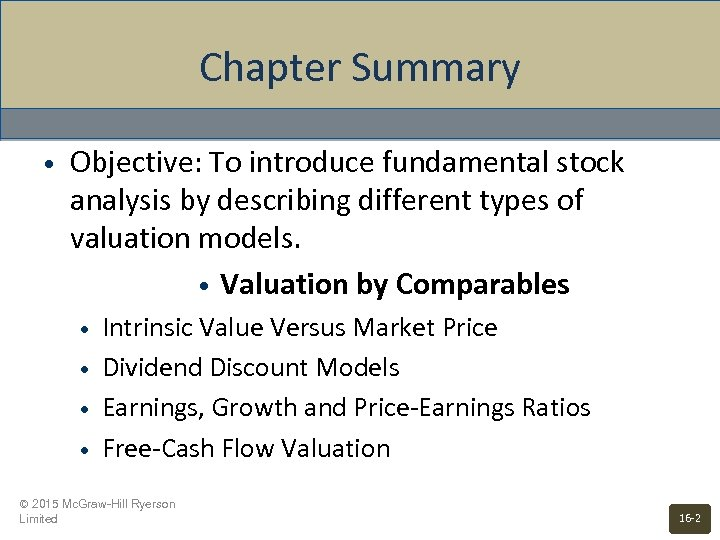Chapter Summary • Objective: To introduce fundamental stock analysis by describing different types of valuation models. • Valuation by Comparables • • Intrinsic Value Versus Market Price Dividend Discount Models Earnings, Growth and Price-Earnings Ratios Free-Cash Flow Valuation © 2015 Mc. Graw-Hill Ryerson Limited 16 -2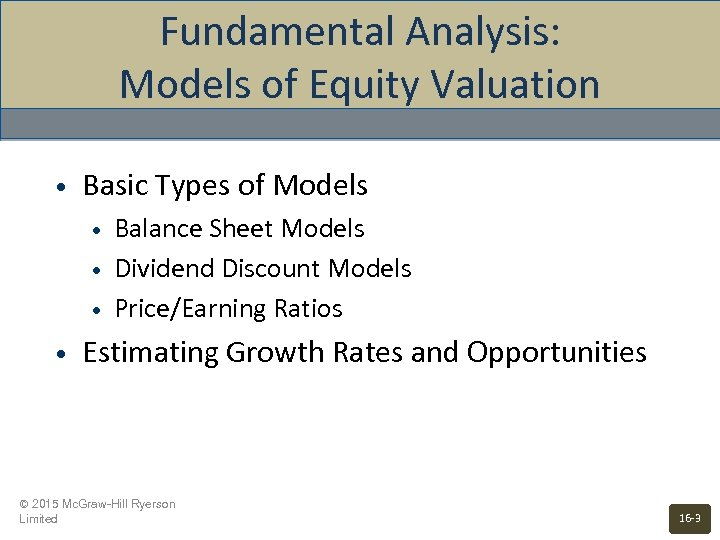Fundamental Analysis: Models of Equity Valuation • Basic Types of Models • • Balance Sheet Models Dividend Discount Models Price/Earning Ratios Estimating Growth Rates and Opportunities © 2015 Mc. Graw-Hill Ryerson Limited 16 -3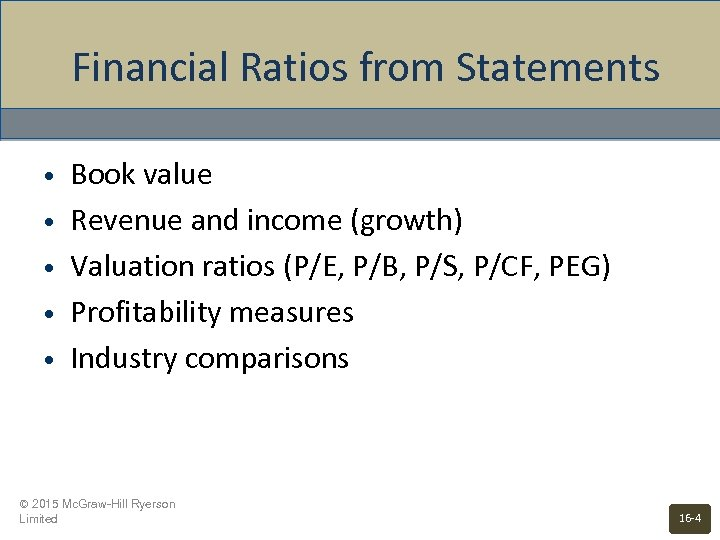Financial Ratios from Statements • • • Book value Revenue and income (growth) Valuation ratios (P/E, P/B, P/S, P/CF, PEG) Profitability measures Industry comparisons © 2015 Mc. Graw-Hill Ryerson Limited 16 -4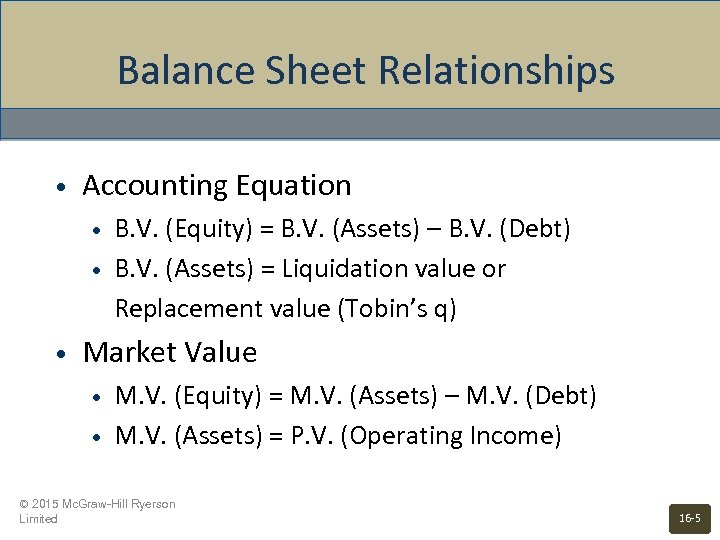Balance Sheet Relationships • Accounting Equation • • • B. V. (Equity) = B. V. (Assets) – B. V. (Debt) B. V. (Assets) = Liquidation value or Replacement value (Tobin’s q) Market Value • • M. V. (Equity) = M. V. (Assets) – M. V. (Debt) M. V. (Assets) = P. V. (Operating Income) © 2015 Mc. Graw-Hill Ryerson Limited 16 -5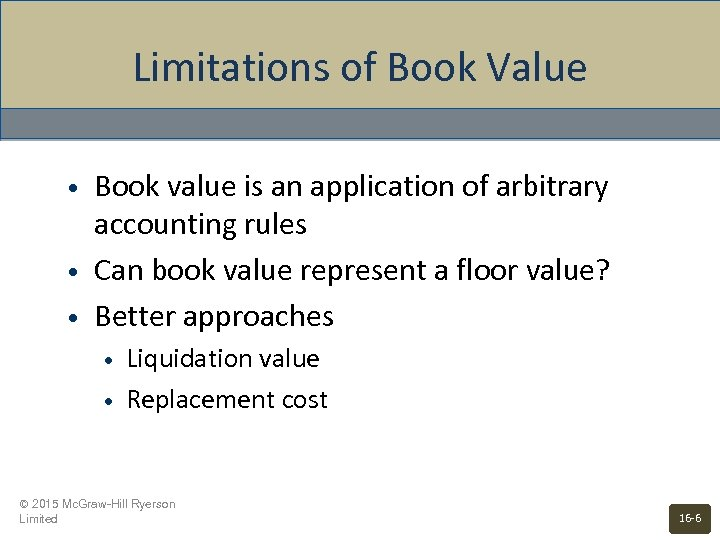Limitations of Book Value • • • Book value is an application of arbitrary accounting rules Can book value represent a floor value? Better approaches • • Liquidation value Replacement cost © 2015 Mc. Graw-Hill Ryerson Limited 16 -6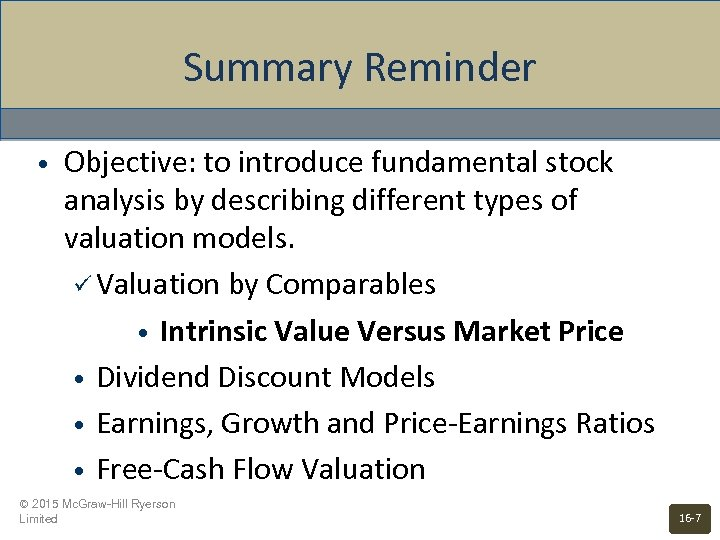Summary Reminder • Objective: to introduce fundamental stock analysis by describing different types of valuation models. ü Valuation by Comparables • Intrinsic Value Versus Market Price • Dividend Discount Models • Earnings, Growth and Price-Earnings Ratios • Free-Cash Flow Valuation © 2015 Mc. Graw-Hill Ryerson Limited 16 -7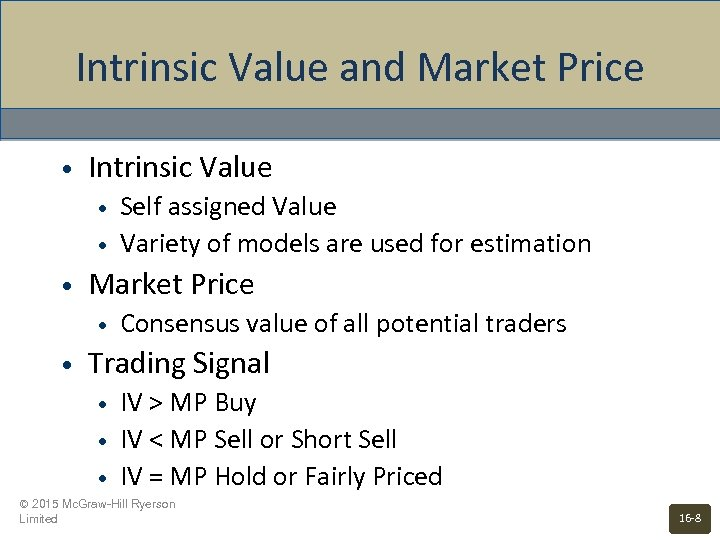Intrinsic Value and Market Price • Intrinsic Value • • • Market Price • • Self assigned Value Variety of models are used for estimation Consensus value of all potential traders Trading Signal • • • IV > MP Buy IV < MP Sell or Short Sell IV = MP Hold or Fairly Priced © 2015 Mc. Graw-Hill Ryerson Limited 16 -8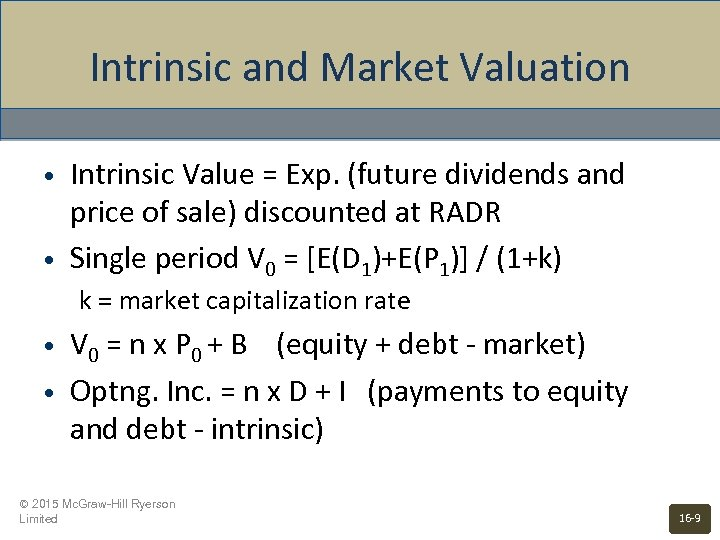Intrinsic and Market Valuation • • Intrinsic Value = Exp. (future dividends and price of sale) discounted at RADR Single period V 0 = [E(D 1)+E(P 1)] / (1+k) k = market capitalization rate • • V 0 = n x P 0 + B (equity + debt - market) Optng. Inc. = n x D + I (payments to equity and debt - intrinsic) © 2015 Mc. Graw-Hill Ryerson Limited 16 -9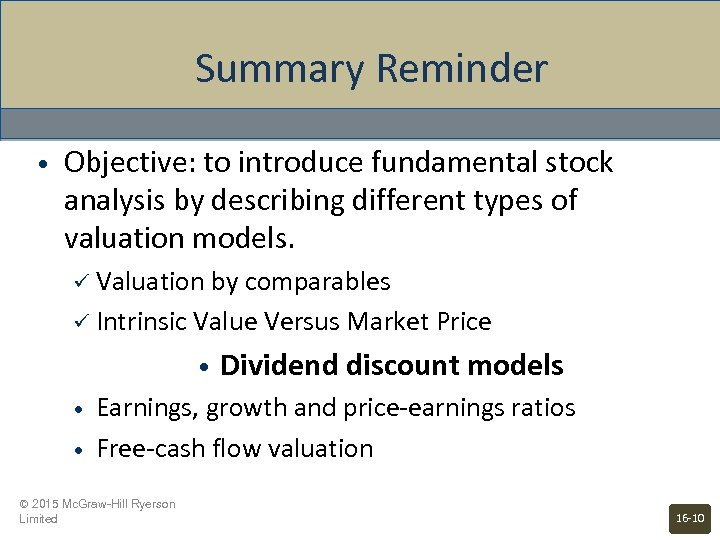Summary Reminder • Objective: to introduce fundamental stock analysis by describing different types of valuation models. ü Valuation by comparables ü Intrinsic Value Versus Market Price • • • Dividend discount models Earnings, growth and price-earnings ratios Free-cash flow valuation © 2015 Mc. Graw-Hill Ryerson Limited 16 -10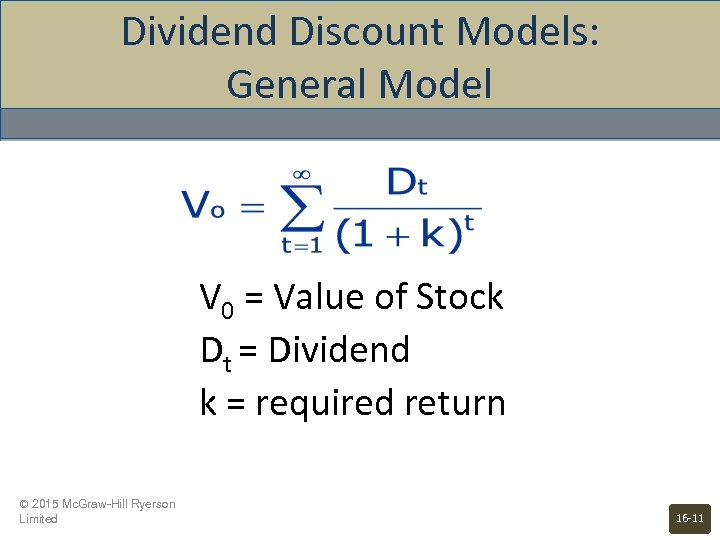Dividend Discount Models: General Model V 0 = Value of Stock Dt = Dividend k = required return © 2015 Mc. Graw-Hill Ryerson Limited 16 -11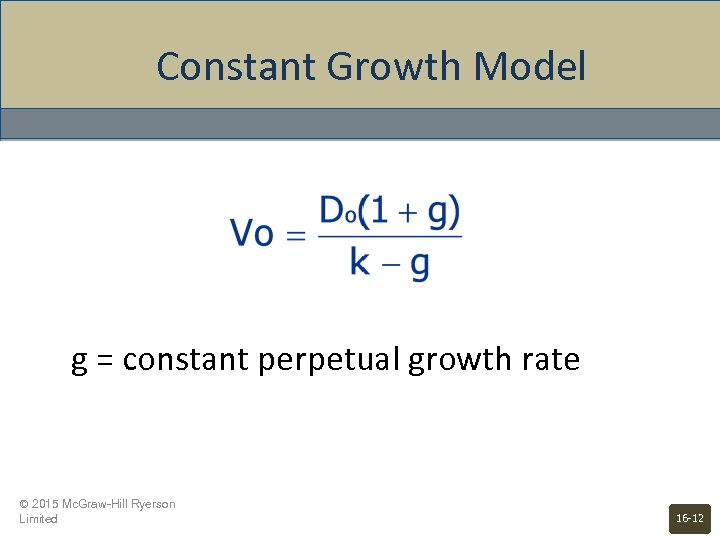Constant Growth Model g = constant perpetual growth rate © 2015 Mc. Graw-Hill Ryerson Limited 16 -12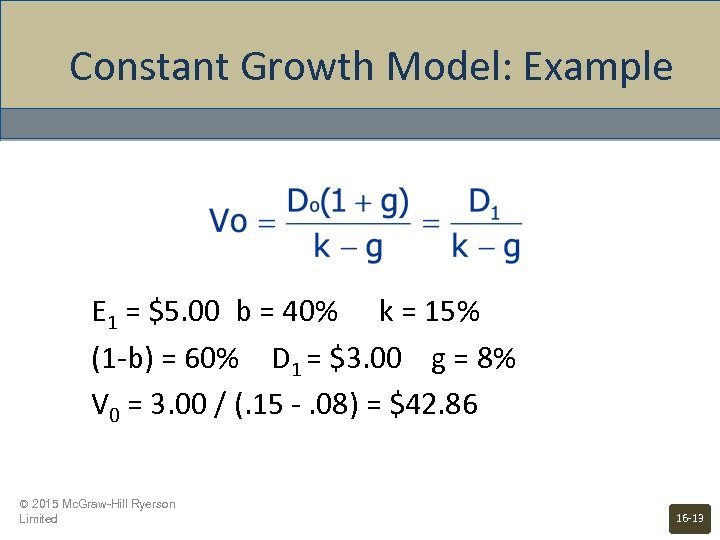Constant Growth Model: Example E 1 = \$5. 00 b = 40% k = 15% (1 -b) = 60% D 1 = \$3. 00 g = 8% V 0 = 3. 00 / (. 15 -. 08) = \$42. 86 © 2015 Mc. Graw-Hill Ryerson Limited 16 -13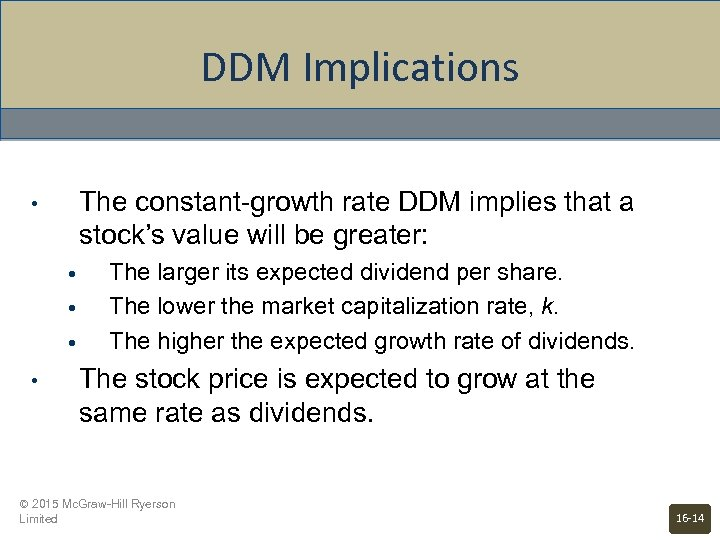DDM Implications The constant-growth rate DDM implies that a stock’s value will be greater: • • • The larger its expected dividend per share. The lower the market capitalization rate, k. The higher the expected growth rate of dividends. The stock price is expected to grow at the same rate as dividends. © 2015 Mc. Graw-Hill Ryerson Limited 16 -14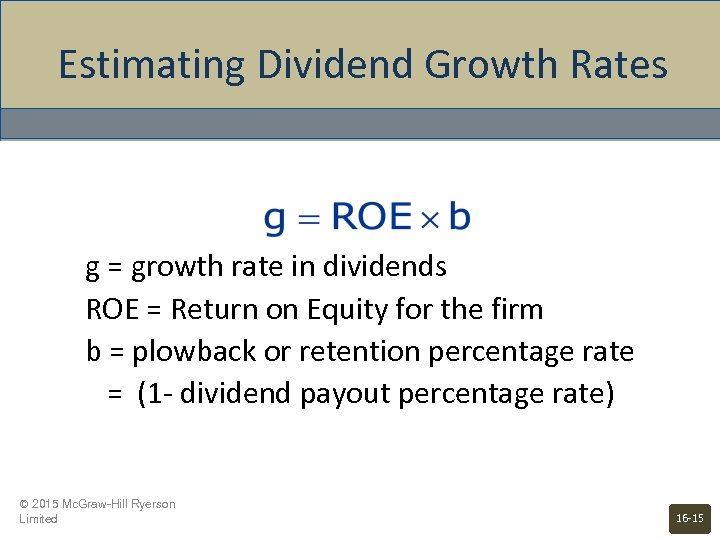Estimating Dividend Growth Rates g = growth rate in dividends ROE = Return on Equity for the firm b = plowback or retention percentage rate = (1 - dividend payout percentage rate) © 2015 Mc. Graw-Hill Ryerson Limited 16 -15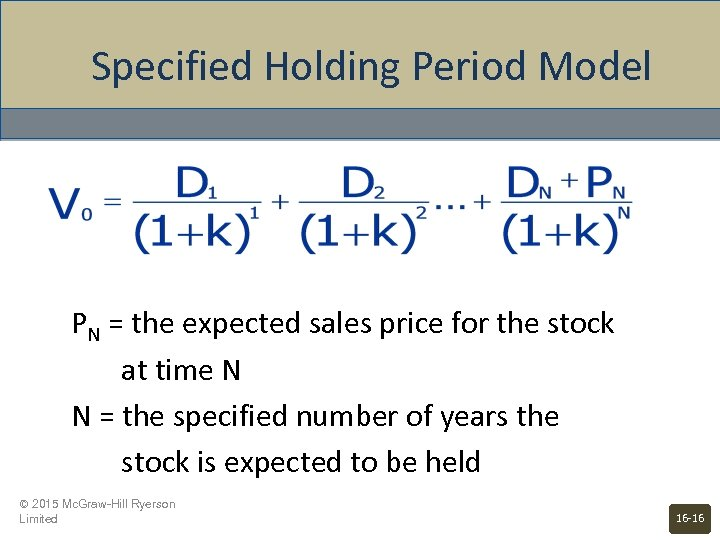Specified Holding Period Model PN = the expected sales price for the stock at time N N = the specified number of years the stock is expected to be held © 2015 Mc. Graw-Hill Ryerson Limited 16 -16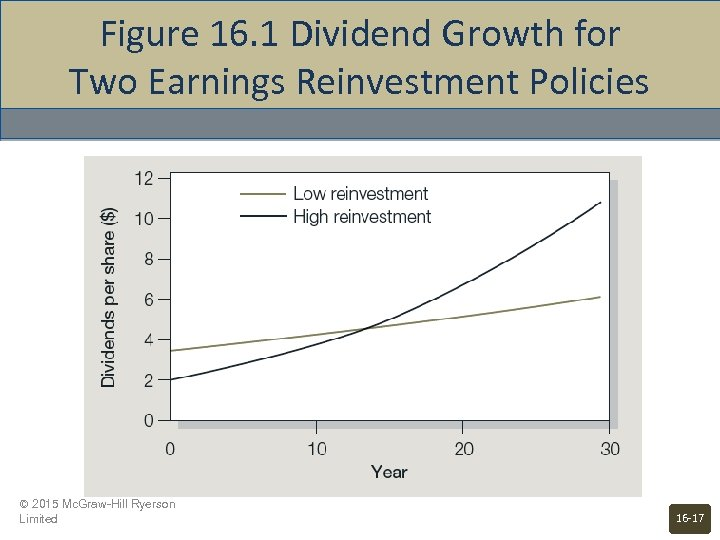Figure 16. 1 Dividend Growth for Two Earnings Reinvestment Policies © 2015 Mc. Graw-Hill Ryerson Limited 16 -17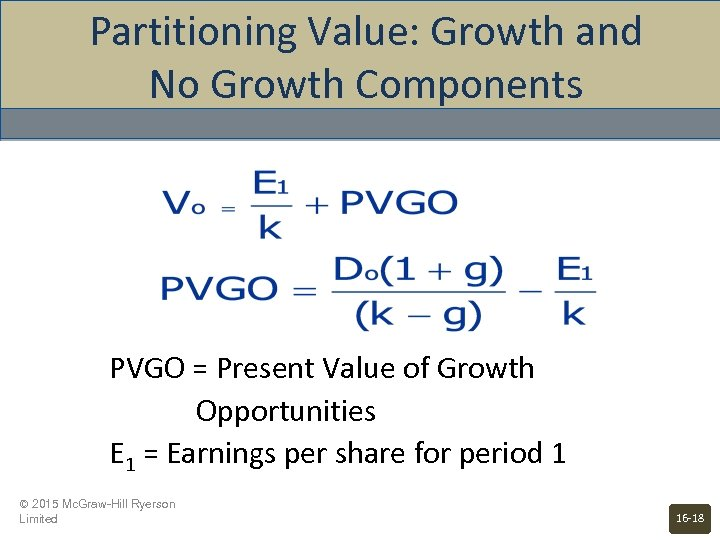Partitioning Value: Growth and No Growth Components PVGO = Present Value of Growth Opportunities E 1 = Earnings per share for period 1 © 2015 Mc. Graw-Hill Ryerson Limited 16 -18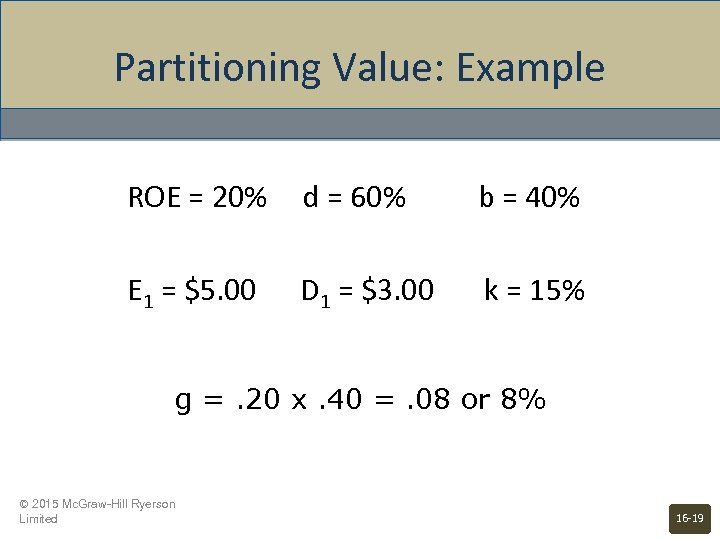Partitioning Value: Example ROE = 20% d = 60% b = 40% E 1 = \$5. 00 D 1 = \$3. 00 k = 15% g =. 20 x. 40 =. 08 or 8% © 2015 Mc. Graw-Hill Ryerson Limited 16 -19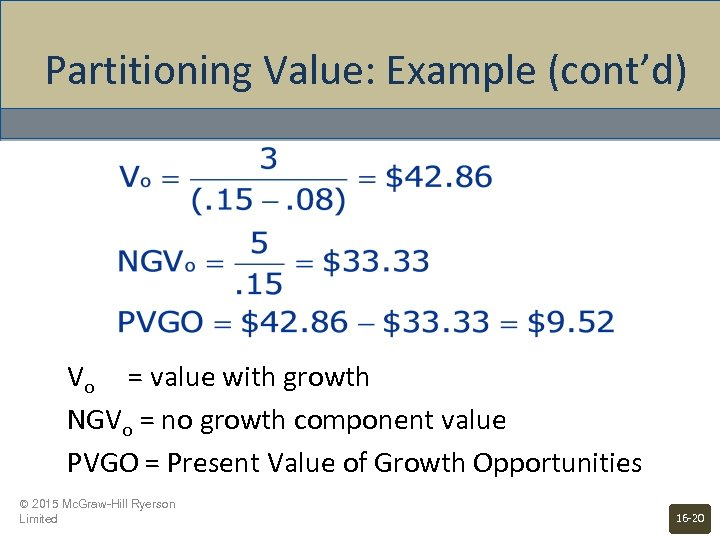Partitioning Value: Example (cont’d) Vo = value with growth NGVo = no growth component value PVGO = Present Value of Growth Opportunities © 2015 Mc. Graw-Hill Ryerson Limited 16 -20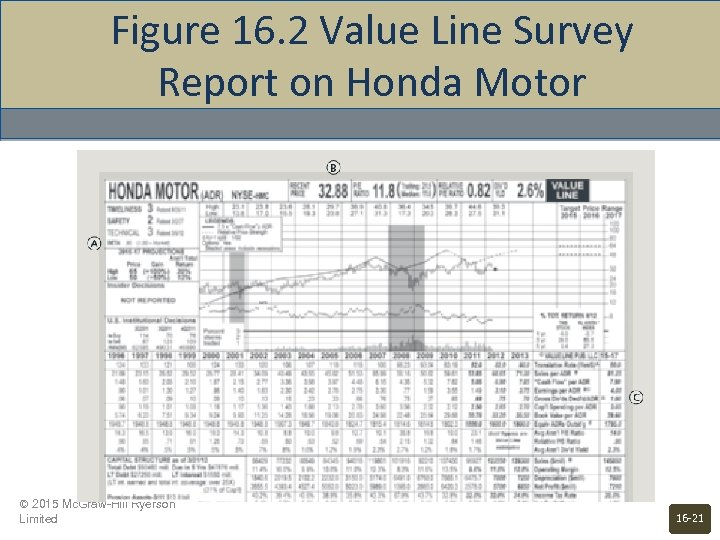Figure 16. 2 Value Line Survey Report on Honda Motor © 2015 Mc. Graw-Hill Ryerson Limited 16 -21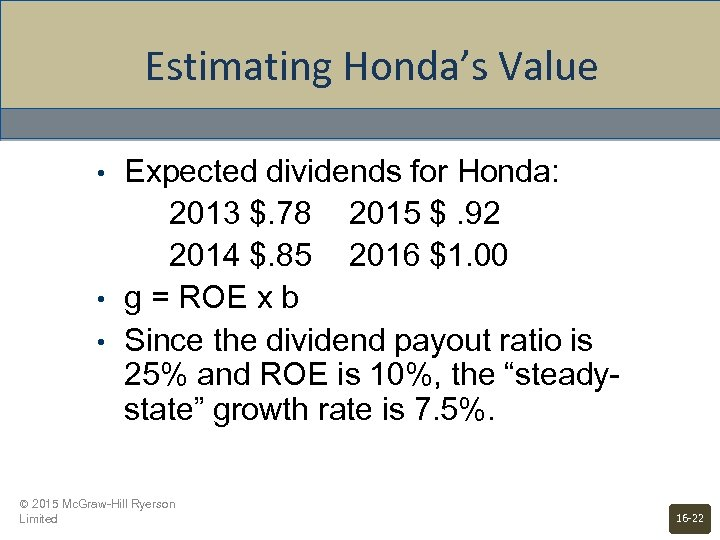Estimating Honda’s Value • • • Expected dividends for Honda: 2013 \$. 78 2015 \$. 92 2014 \$. 85 2016 \$1. 00 g = ROE x b Since the dividend payout ratio is 25% and ROE is 10%, the “steadystate” growth rate is 7. 5%. © 2015 Mc. Graw-Hill Ryerson Limited 16 -22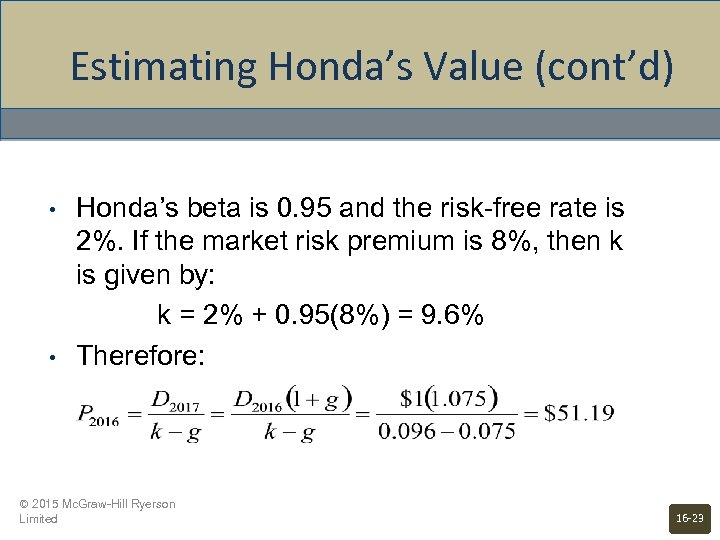Estimating Honda’s Value (cont’d) • • Honda’s beta is 0. 95 and the risk-free rate is 2%. If the market risk premium is 8%, then k is given by: k = 2% + 0. 95(8%) = 9. 6% Therefore: © 2015 Mc. Graw-Hill Ryerson Limited 16 -23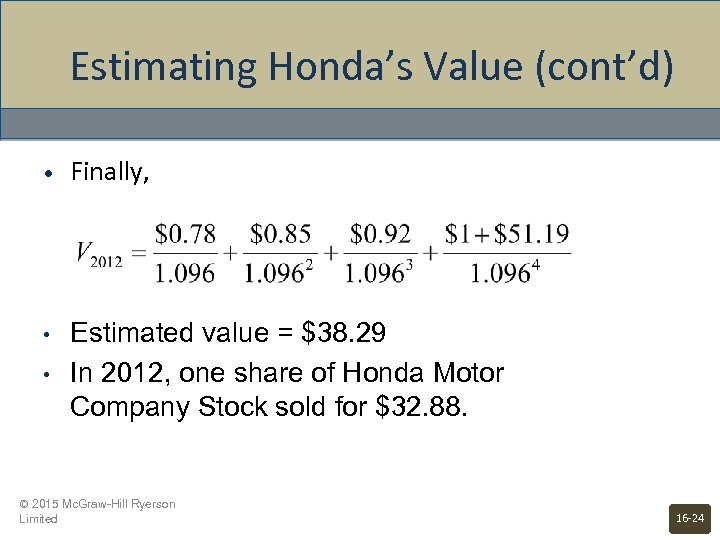Estimating Honda’s Value (cont’d) • Finally, • Estimated value = \$38. 29 In 2012, one share of Honda Motor Company Stock sold for \$32. 88. • © 2015 Mc. Graw-Hill Ryerson Limited 16 -24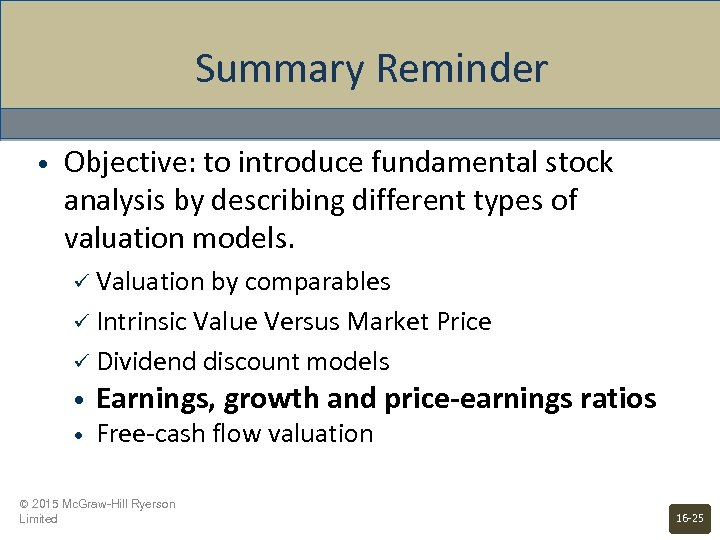Summary Reminder • Objective: to introduce fundamental stock analysis by describing different types of valuation models. ü Valuation by comparables ü Intrinsic Value Versus Market Price ü Dividend discount models • • Earnings, growth and price-earnings ratios Free-cash flow valuation © 2015 Mc. Graw-Hill Ryerson Limited 16 -25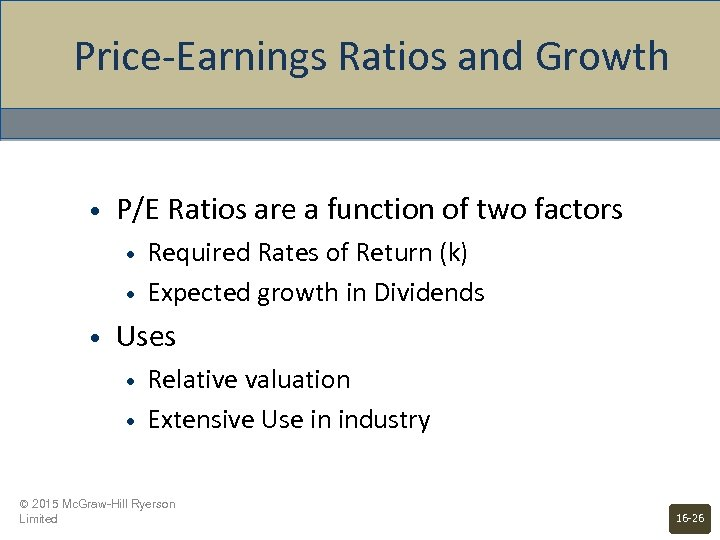Price-Earnings Ratios and Growth • P/E Ratios are a function of two factors • • • Required Rates of Return (k) Expected growth in Dividends Uses • • Relative valuation Extensive Use in industry © 2015 Mc. Graw-Hill Ryerson Limited 16 -26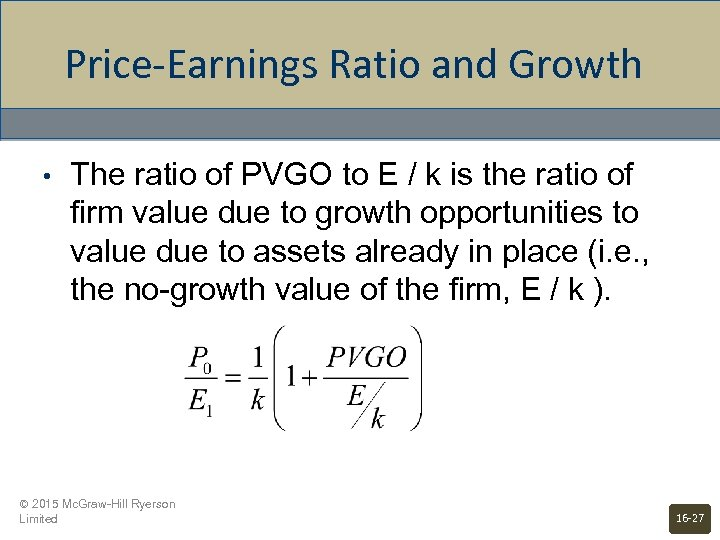Price-Earnings Ratio and Growth • The ratio of PVGO to E / k is the ratio of firm value due to growth opportunities to value due to assets already in place (i. e. , the no-growth value of the firm, E / k ). © 2015 Mc. Graw-Hill Ryerson Limited 16 -27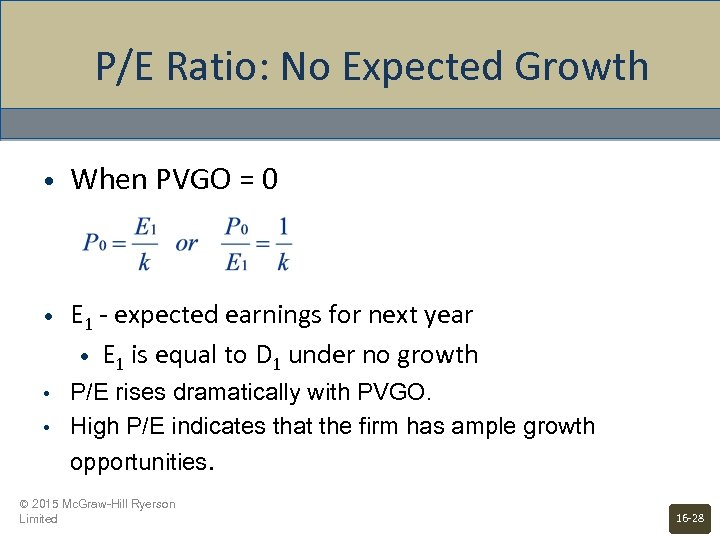P/E Ratio: No Expected Growth • When PVGO = 0 • E 1 - expected earnings for next year • E 1 is equal to D 1 under no growth • P/E rises dramatically with PVGO. High P/E indicates that the firm has ample growth opportunities. • © 2015 Mc. Graw-Hill Ryerson Limited 16 -28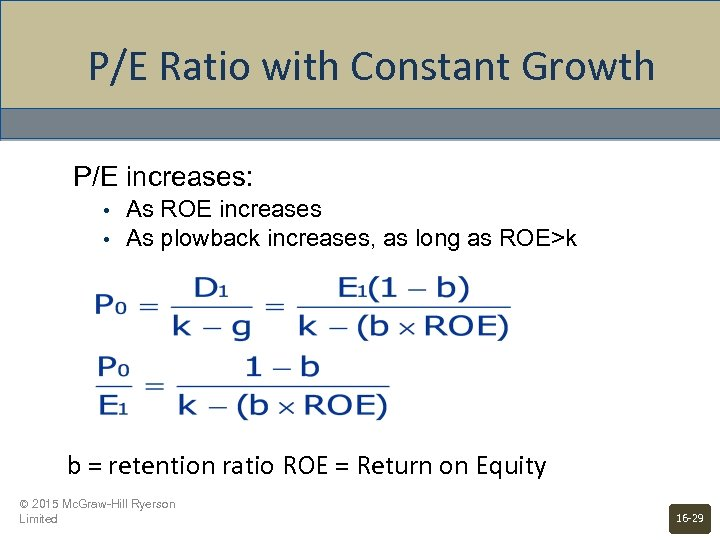P/E Ratio with Constant Growth P/E increases: • • As ROE increases As plowback increases, as long as ROE>k b = retention ratio ROE = Return on Equity © 2015 Mc. Graw-Hill Ryerson Limited 16 -29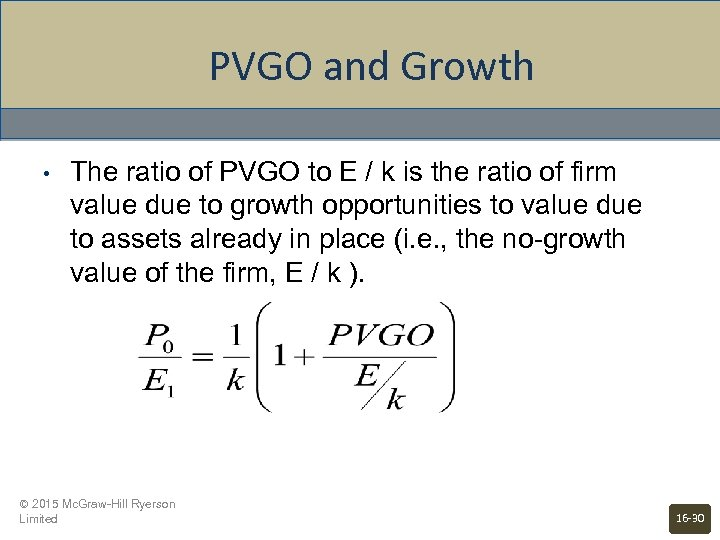PVGO and Growth • The ratio of PVGO to E / k is the ratio of firm value due to growth opportunities to value due to assets already in place (i. e. , the no-growth value of the firm, E / k ). © 2015 Mc. Graw-Hill Ryerson Limited 16 -30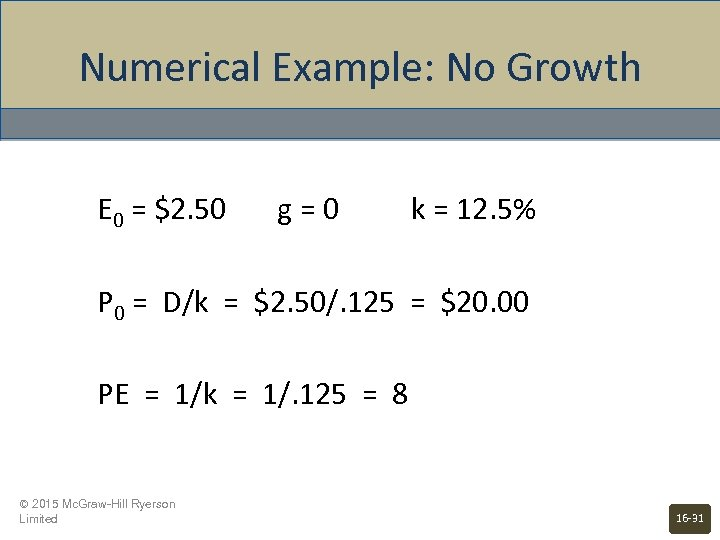Numerical Example: No Growth E 0 = \$2. 50 g=0 k = 12. 5% P 0 = D/k = \$2. 50/. 125 = \$20. 00 PE = 1/k = 1/. 125 = 8 © 2015 Mc. Graw-Hill Ryerson Limited 16 -31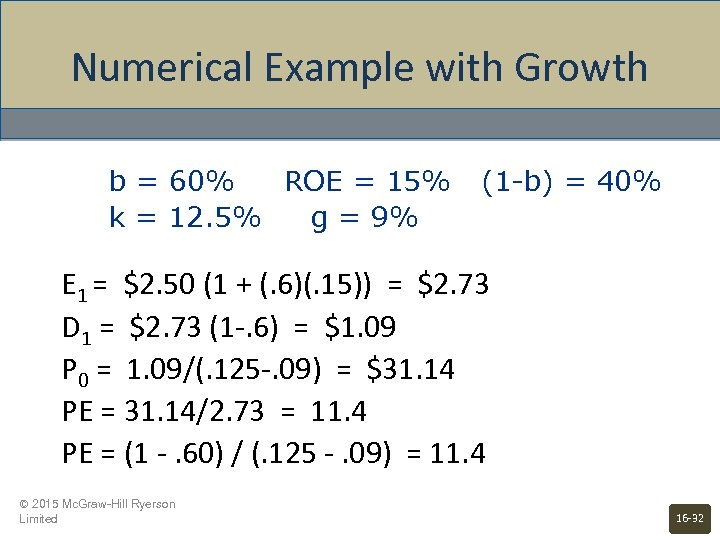Numerical Example with Growth b = 60% ROE = 15% k = 12. 5% g = 9% (1 -b) = 40% E 1 = \$2. 50 (1 + (. 6)(. 15)) = \$2. 73 D 1 = \$2. 73 (1 -. 6) = \$1. 09 P 0 = 1. 09/(. 125 -. 09) = \$31. 14 PE = 31. 14/2. 73 = 11. 4 PE = (1 -. 60) / (. 125 -. 09) = 11. 4 © 2015 Mc. Graw-Hill Ryerson Limited 16 -32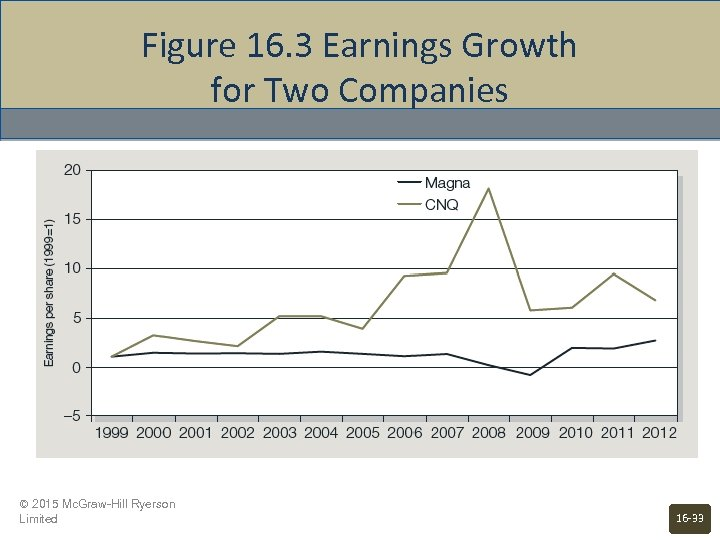Figure 16. 3 Earnings Growth for Two Companies © 2015 Mc. Graw-Hill Ryerson Limited 16 -33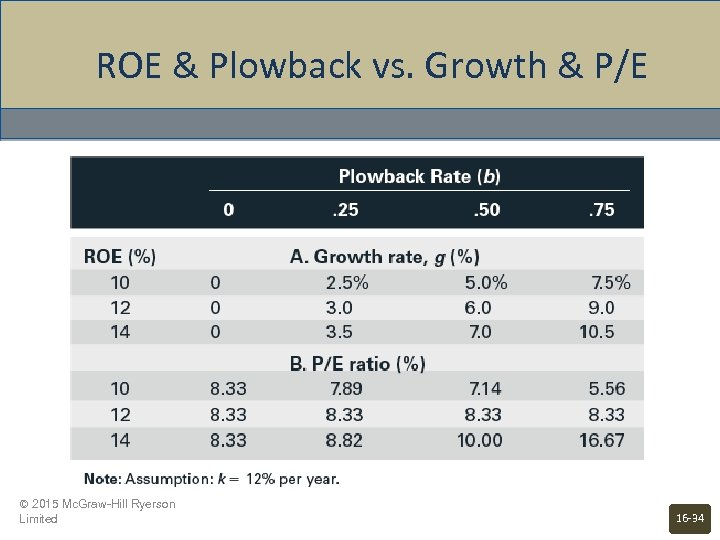ROE & Plowback vs. Growth & P/E © 2015 Mc. Graw-Hill Ryerson Limited 16 -34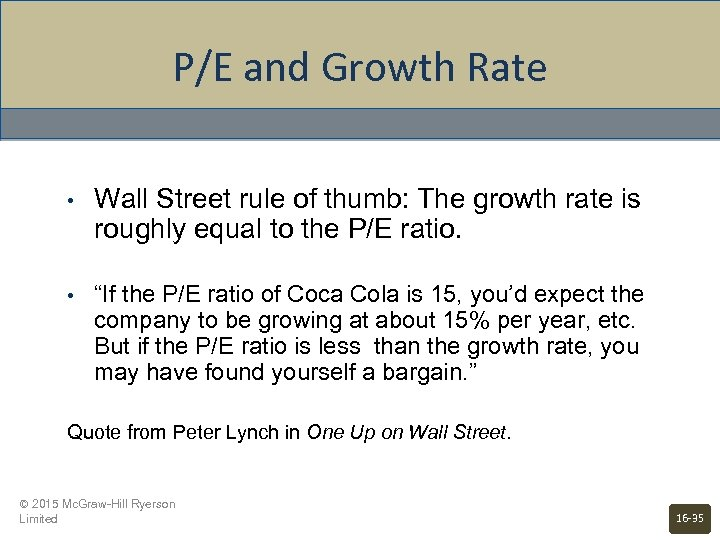P/E and Growth Rate • Wall Street rule of thumb: The growth rate is roughly equal to the P/E ratio. • “If the P/E ratio of Coca Cola is 15, you’d expect the company to be growing at about 15% per year, etc. But if the P/E ratio is less than the growth rate, you may have found yourself a bargain. ” Quote from Peter Lynch in One Up on Wall Street. © 2015 Mc. Graw-Hill Ryerson Limited 16 -35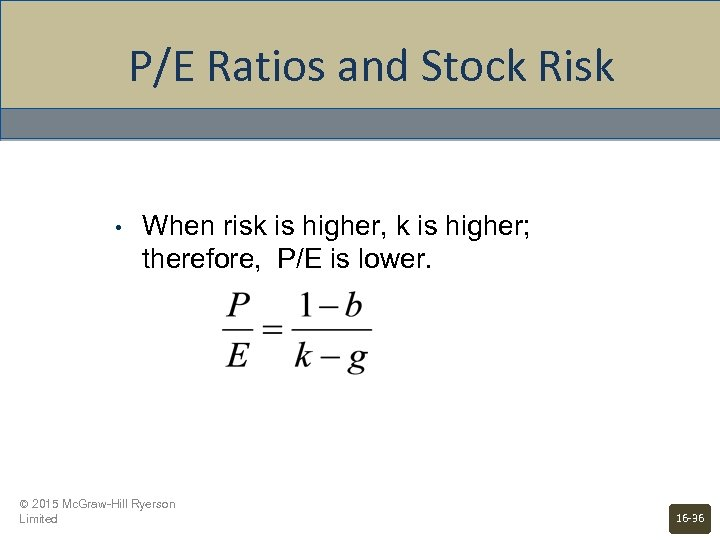P/E Ratios and Stock Risk • When risk is higher, k is higher; therefore, P/E is lower. © 2015 Mc. Graw-Hill Ryerson Limited 16 -36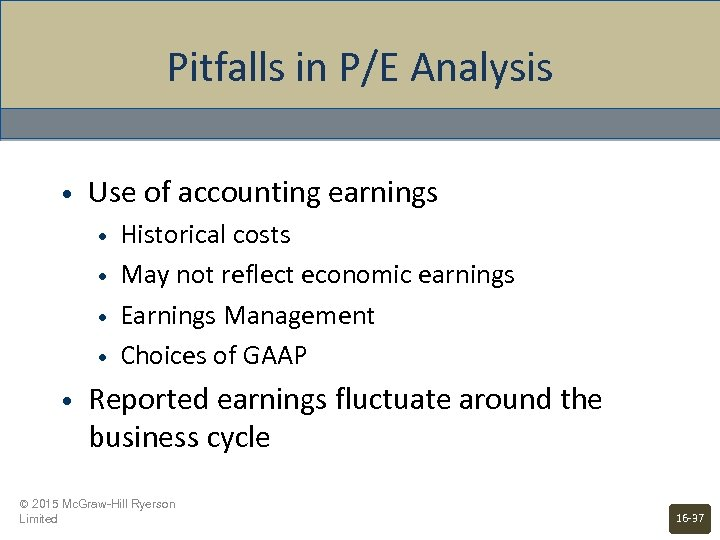Pitfalls in P/E Analysis • Use of accounting earnings • • • Historical costs May not reflect economic earnings Earnings Management Choices of GAAP Reported earnings fluctuate around the business cycle © 2015 Mc. Graw-Hill Ryerson Limited 16 -37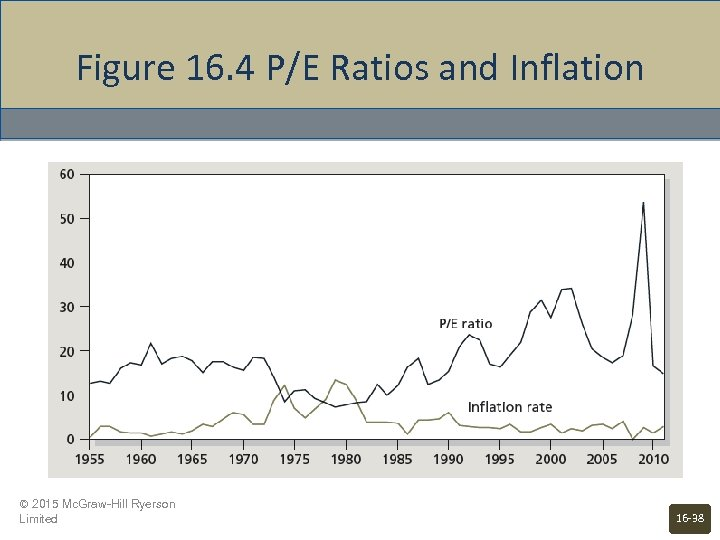Figure 16. 4 P/E Ratios and Inflation © 2015 Mc. Graw-Hill Ryerson Limited 16 -38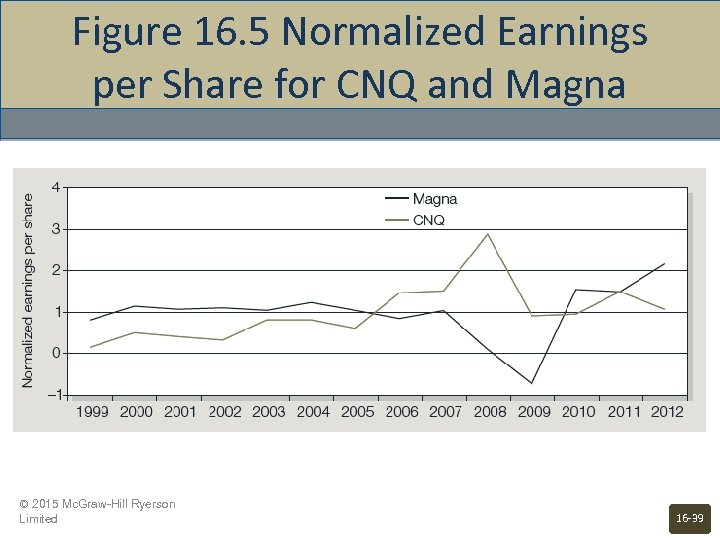Figure 16. 5 Normalized Earnings per Share for CNQ and Magna © 2015 Mc. Graw-Hill Ryerson Limited 16 -39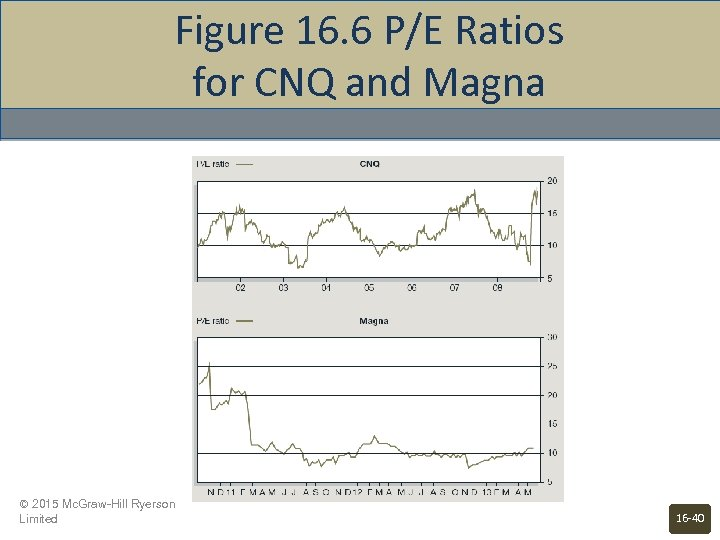Figure 16. 6 P/E Ratios for CNQ and Magna © 2015 Mc. Graw-Hill Ryerson Limited 16 -40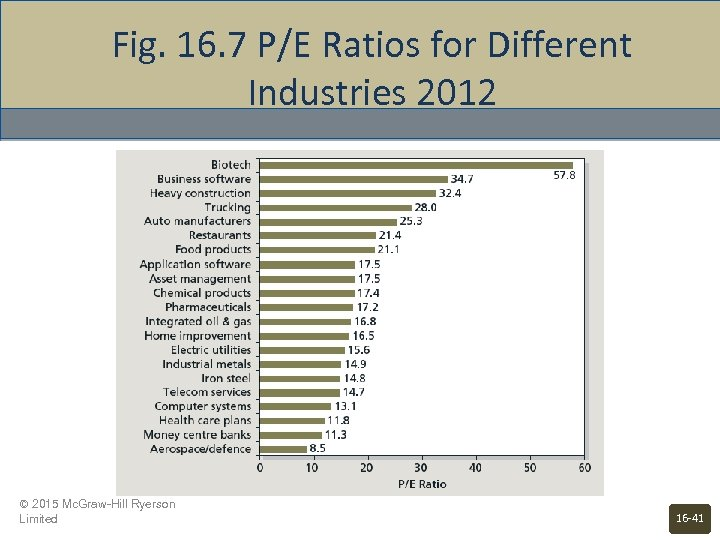Fig. 16. 7 P/E Ratios for Different Industries 2012 © 2015 Mc. Graw-Hill Ryerson Limited 16 -41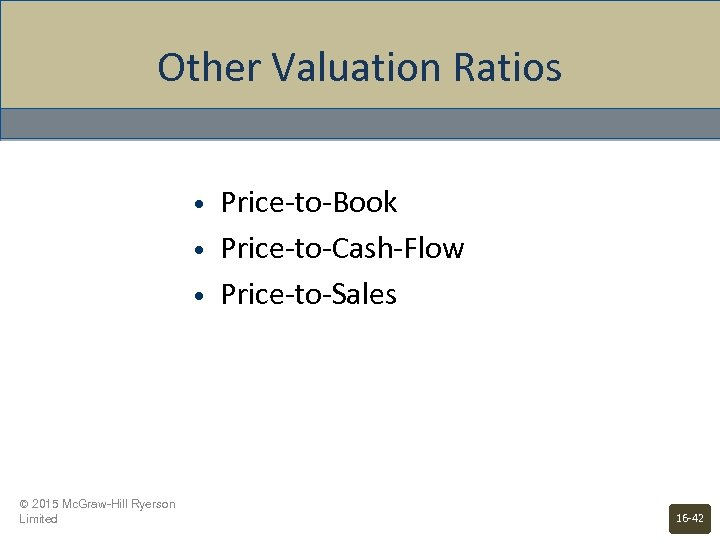Other Valuation Ratios • • • © 2015 Mc. Graw-Hill Ryerson Limited Price-to-Book Price-to-Cash-Flow Price-to-Sales 16 -42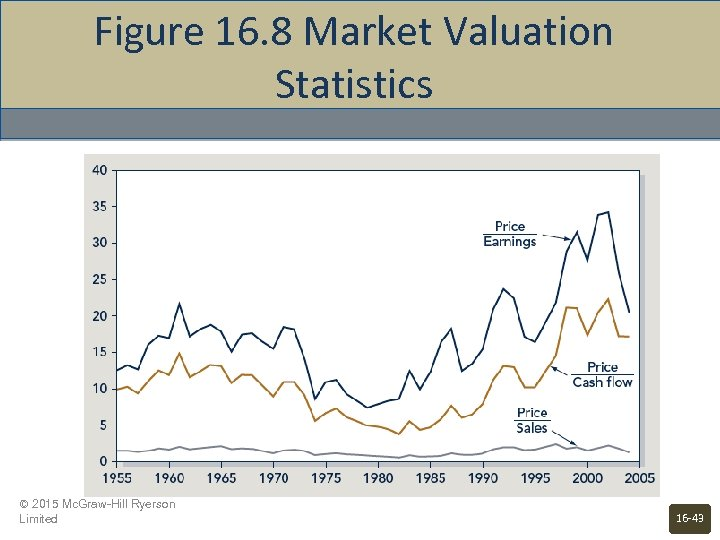Figure 16. 8 Market Valuation Statistics © 2015 Mc. Graw-Hill Ryerson Limited 16 -43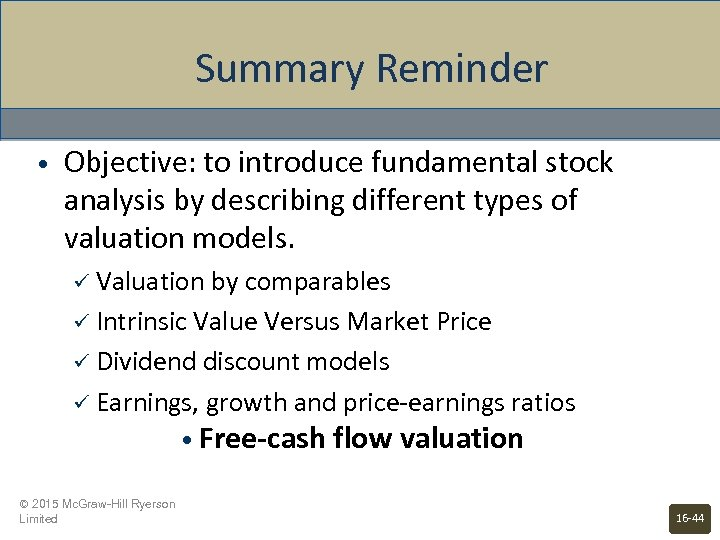Summary Reminder • Objective: to introduce fundamental stock analysis by describing different types of valuation models. ü Valuation by comparables ü Intrinsic Value Versus Market Price ü Dividend discount models ü Earnings, growth and price-earnings ratios • Free-cash flow valuation © 2015 Mc. Graw-Hill Ryerson Limited 16 -44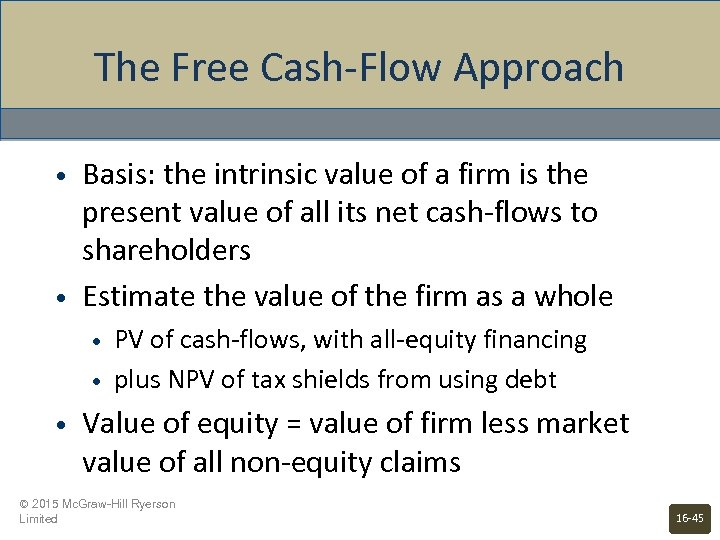The Free Cash-Flow Approach • • Basis: the intrinsic value of a firm is the present value of all its net cash-flows to shareholders Estimate the value of the firm as a whole • • • PV of cash-flows, with all-equity financing plus NPV of tax shields from using debt Value of equity = value of firm less market value of all non-equity claims © 2015 Mc. Graw-Hill Ryerson Limited 16 -45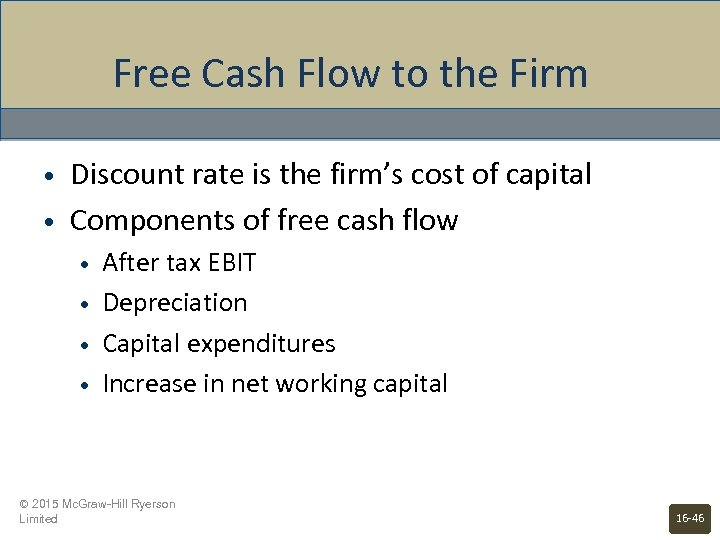Free Cash Flow to the Firm • • Discount rate is the firm’s cost of capital Components of free cash flow • • After tax EBIT Depreciation Capital expenditures Increase in net working capital © 2015 Mc. Graw-Hill Ryerson Limited 16 -46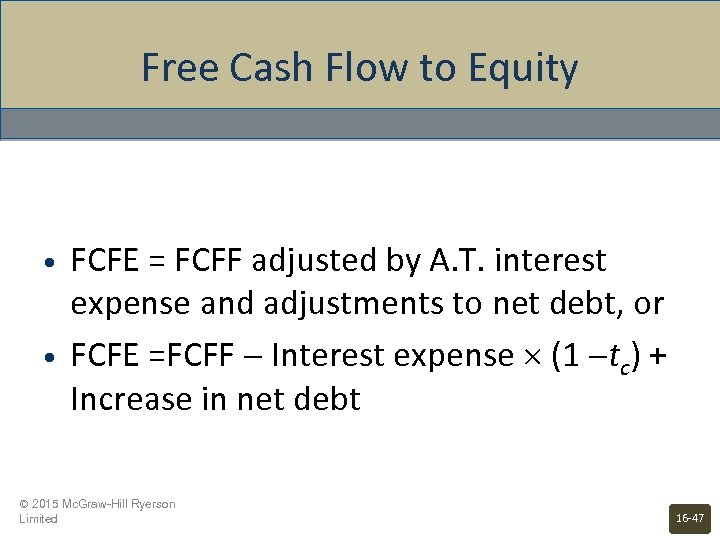Free Cash Flow to Equity • • FCFE = FCFF adjusted by A. T. interest expense and adjustments to net debt, or FCFE =FCFF Interest expense (1 tc) + Increase in net debt © 2015 Mc. Graw-Hill Ryerson Limited 16 -47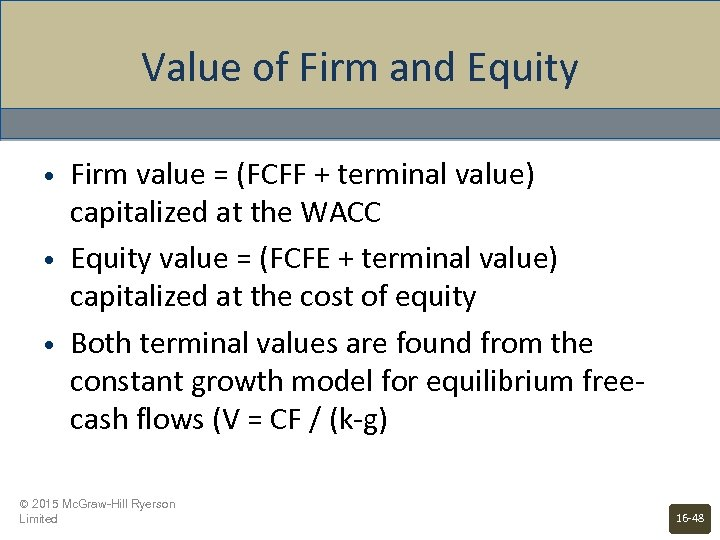Value of Firm and Equity • • • Firm value = (FCFF + terminal value) capitalized at the WACC Equity value = (FCFE + terminal value) capitalized at the cost of equity Both terminal values are found from the constant growth model for equilibrium freecash flows (V = CF / (k-g) © 2015 Mc. Graw-Hill Ryerson Limited 16 -48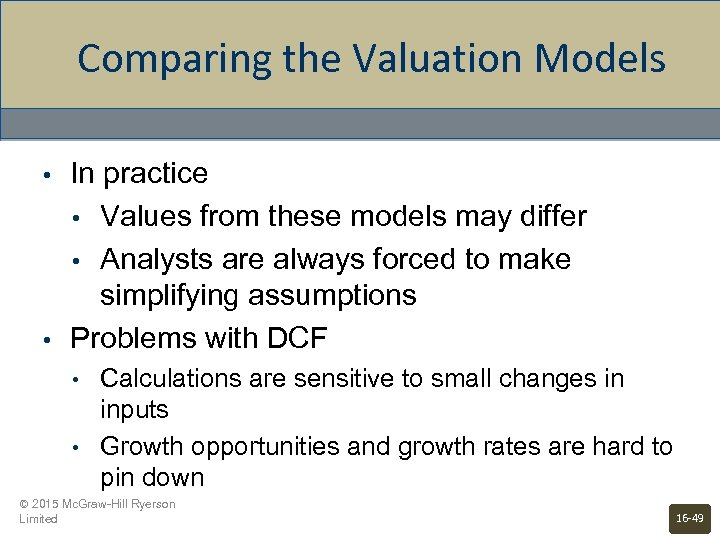Comparing the Valuation Models • • In practice • Values from these models may differ • Analysts are always forced to make simplifying assumptions Problems with DCF • • Calculations are sensitive to small changes in inputs Growth opportunities and growth rates are hard to pin down © 2015 Mc. Graw-Hill Ryerson Limited 16 -49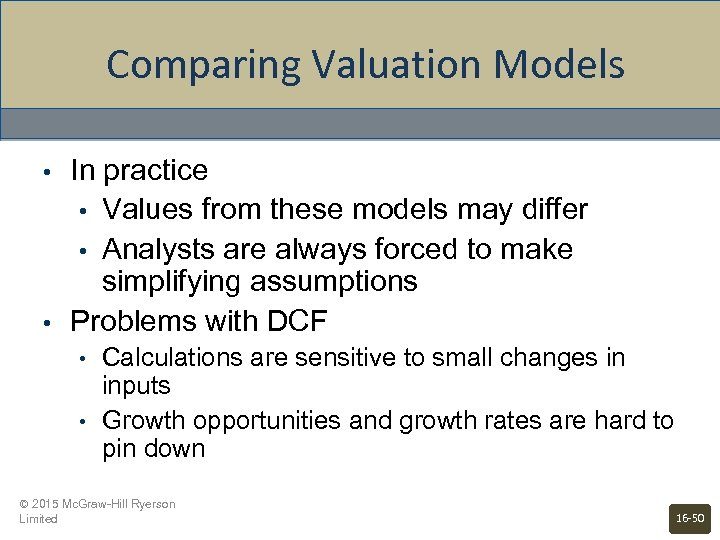Comparing Valuation Models • • In practice • Values from these models may differ • Analysts are always forced to make simplifying assumptions Problems with DCF • • Calculations are sensitive to small changes in inputs Growth opportunities and growth rates are hard to pin down © 2015 Mc. Graw-Hill Ryerson Limited 16 -50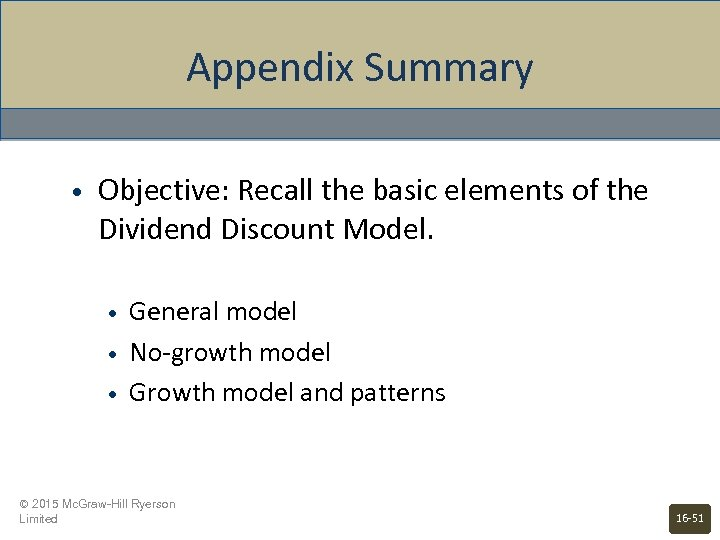Appendix Summary • Objective: Recall the basic elements of the Dividend Discount Model. • • • General model No-growth model Growth model and patterns © 2015 Mc. Graw-Hill Ryerson Limited 16 -51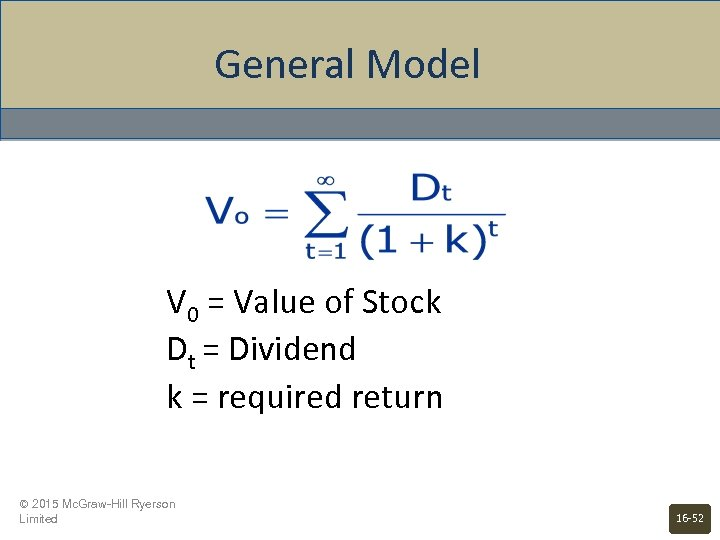General Model V 0 = Value of Stock Dt = Dividend k = required return © 2015 Mc. Graw-Hill Ryerson Limited 16 -52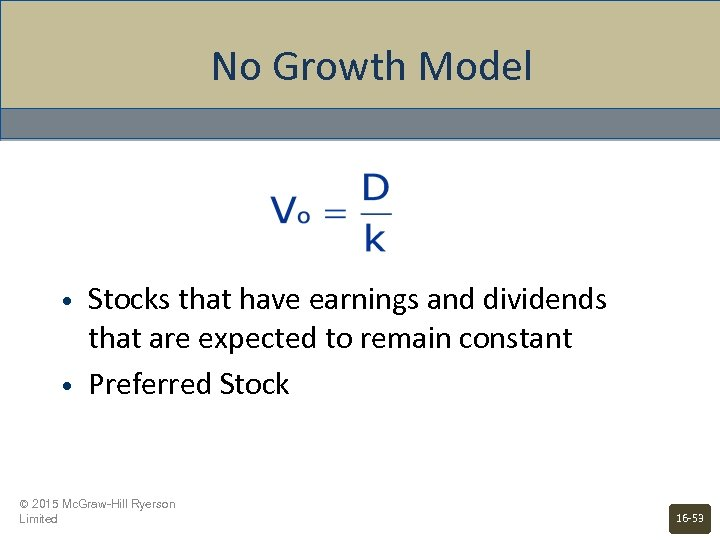No Growth Model • • Stocks that have earnings and dividends that are expected to remain constant Preferred Stock © 2015 Mc. Graw-Hill Ryerson Limited 16 -53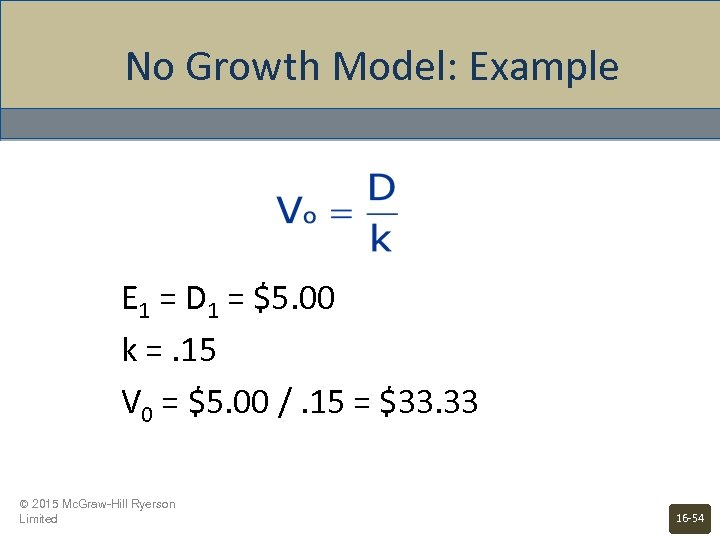No Growth Model: Example E 1 = D 1 = \$5. 00 k =. 15 V 0 = \$5. 00 /. 15 = \$33. 33 © 2015 Mc. Graw-Hill Ryerson Limited 16 -54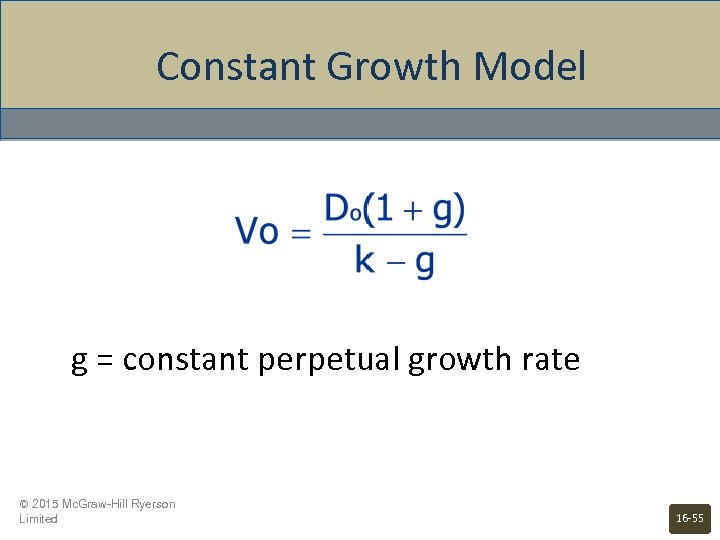Constant Growth Model g = constant perpetual growth rate © 2015 Mc. Graw-Hill Ryerson Limited 16 -55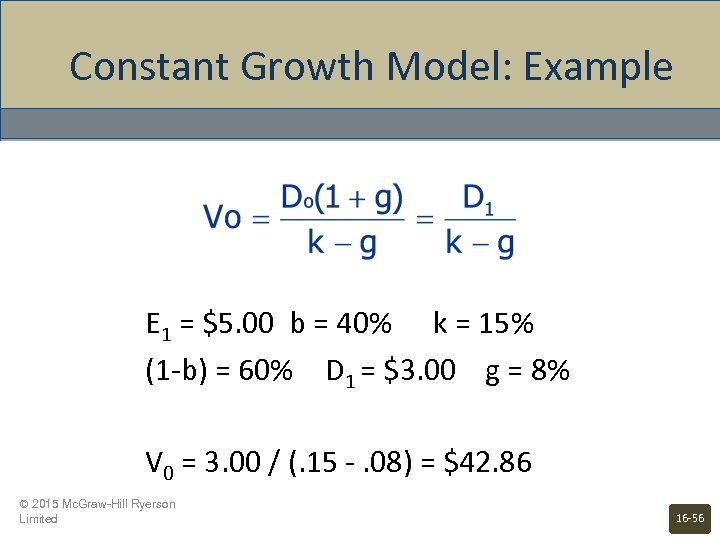Constant Growth Model: Example E 1 = \$5. 00 b = 40% k = 15% (1 -b) = 60% D 1 = \$3. 00 g = 8% V 0 = 3. 00 / (. 15 -. 08) = \$42. 86 © 2015 Mc. Graw-Hill Ryerson Limited 16 -56